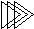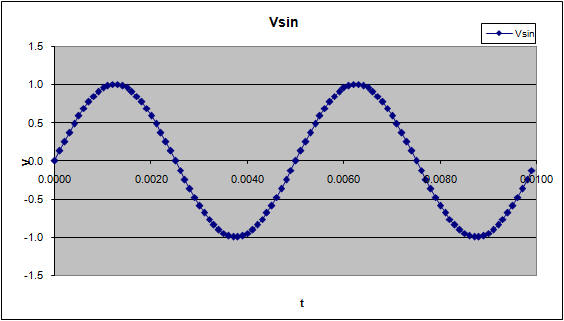Electronic Design with Excel
 Topics | VBA Basics | Back to eCircuit Center

SINE WAVE GENERATOR

SIGNAL GENERATOR

Here's a way to generate and explore the sine wave . You can change its frequency, offset, phase and see the wave form change. The VBA function is a simple equation. To see the VBA code hit ALT-F11 and double click on the Modules > Module1 in the VBA Project window. This opens the code window for this module.

 Function SineWave(t, Vp, fo, Phase, Vdc)   ' create sine wave   ' phase in deg   Dim pi As Double   pi = 3.1415927   'Calc sine wave   SineWave = Vp * Sin(2 * pi * fo * t + Phase * pi / 180) + Vdc End Function

The function calculates the value of the of the sine wave at each time point t, given the

Vp       - Magnitude Peak (V)
fo        - Frequency (Hz)
Phase - Phase (deg)
Vdc     - Offset Voltage (V)

The phase gets converted from degrees to radians inside the SIn function as
Phase * pi / 180

AMPLITUDE VERSUS TIME

You enter the description of the sine wave in these cells.

 Vp 1 V VDC 0 V fo 200 Hz Phase 0 deg dT 0.0001

The time column is generated by entering the time increment dT at location C14. Each time point is simply the previous time point plus the delta, A17+\$C\$14. Note, that C14 is a fixed reference point.

 time Vsin 0.0000 0.00 0.0001 0.06 0.0002 0.13 0.0003 0.19 0.0004 0.25 0.0005 0.31 0.0006 0.37 0.0007 0.43 0.0008 0.48 0.0009 0.54

The cells in the Vsin column holds the function call. The first entry in this column looks like

=SineWave(A17,\$C\$9,\$C\$11,\$C\$12,\$C\$10)

where A17 is the current time point and C9 through C10 hold the sine parameters. The fun part of this spreadsheet is changing the parameters and then watching the plot change.